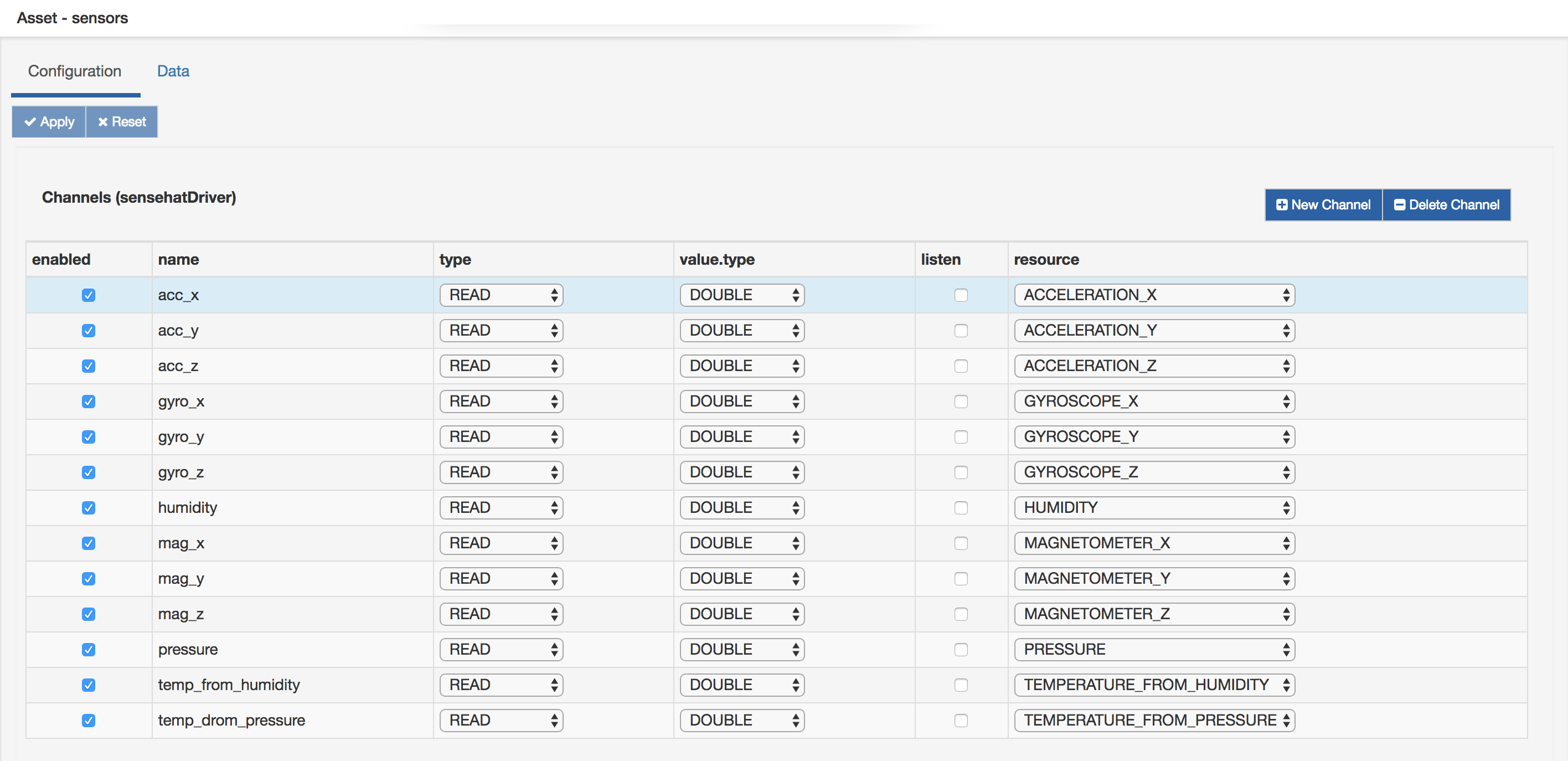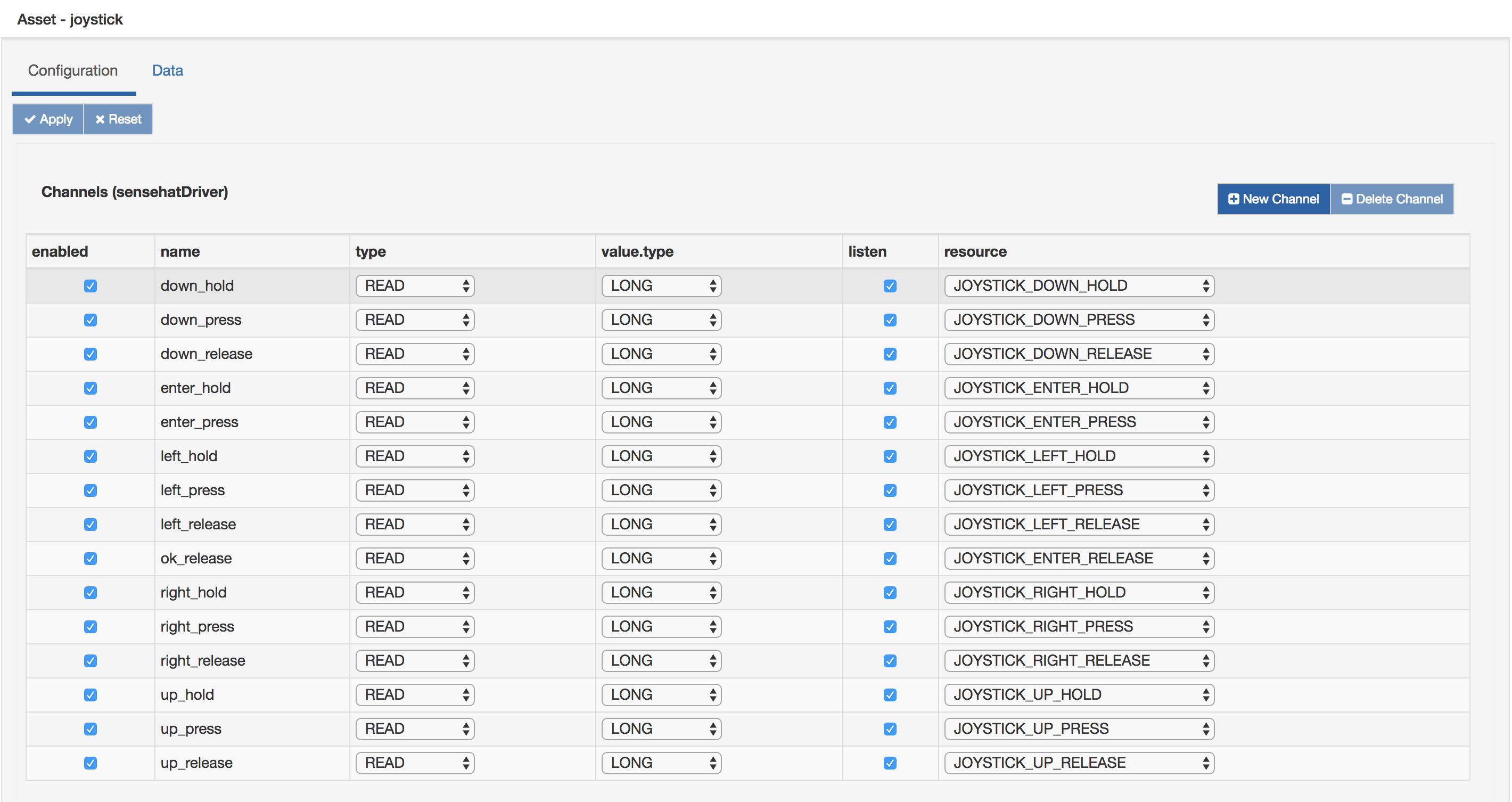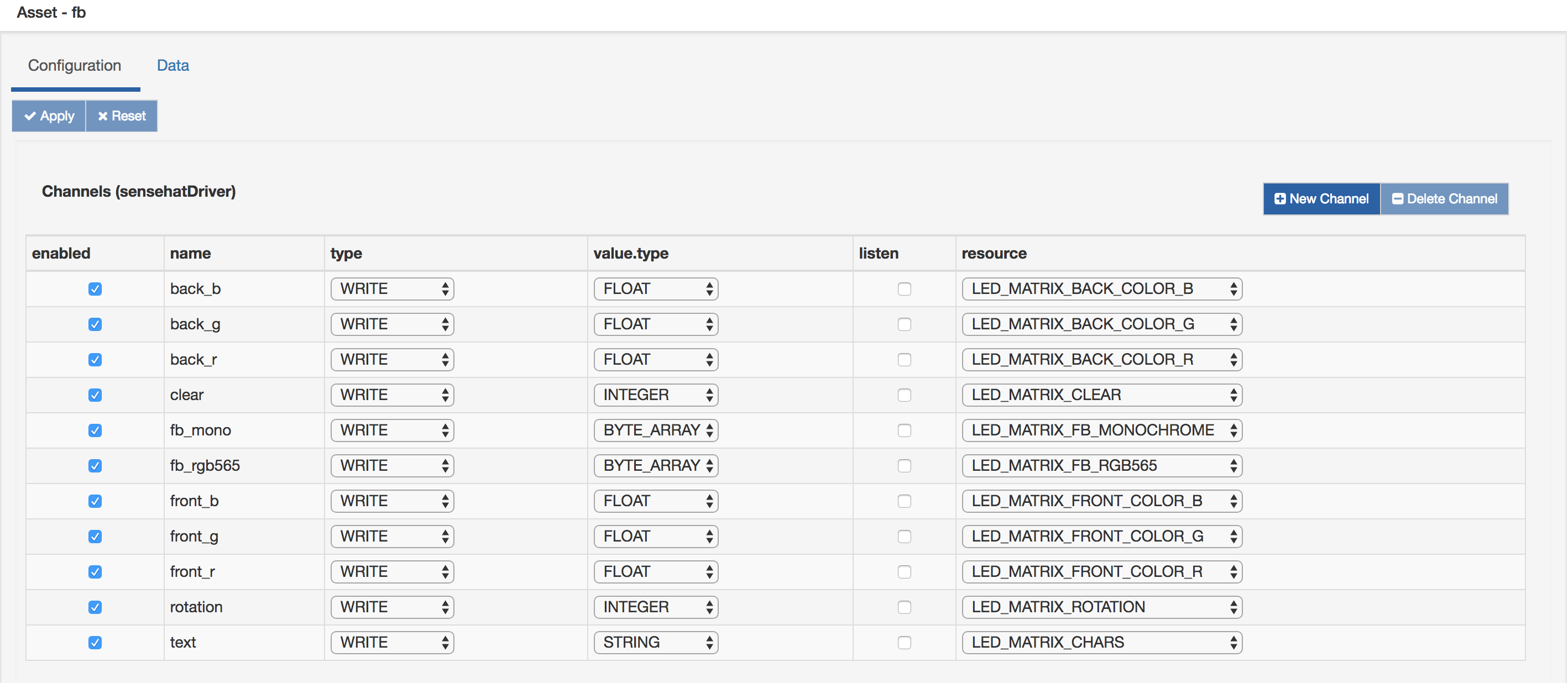# SenseHat Driver

The SenseHat driver allows to interact to a RaspberryPi SenseHat device using Kura Driver, Asset and Wires frameworks.

The driver-specific channel configuration contains a single parameter, resource, which allows to select the specific device resource that the channel is addressing (a sensor, a joystick event, etc).

## Sensors

The following values of the resource parameters refer to device sensors:

ResourceUnitDescription
ACCELERATION_X, ACCELERATION_Y, ACCELERATION_ZGThe proper acceleration for each axis
GYROSCOPE_X, GYROSCOPE_Y, GYROSCOPE_Zrad/SThe angular acceleration for each axis
MAGNETOMETER_X, MAGNETOMETER_Y, MAGNETOMETERE_ZuTThe magnetometer value for each axis
HUMIDITY%rHThe relative humidity
PRESSUREmbarThe pressure value
TEMPERATURE_FROM_HUMIDITY°CThe temperature obtained from the humidity sensor
TEMPERATURE_FROM_PRESSURE°CThe temperature obtained from the pressure sensor

The channels referencing sensor resources can only be used for reads and only in polling mode.
The driver will attempt to convert the value obtained from the sensor into the data type selected using the value.type parameter

Example sensors Asset configuration:## Joystick

The SenseHat joystick provides four buttons:

• UP
• DOWN
• LEFT
• RIGHT
• ENTER

For each button, the driver allows to listen to the following events:

• PRESS: Fired once when a button is pressed
• RELEASE: Fired once when a button is released
• HOLD: Fired periodically every few seconds if the button is kept pressed

The values of the resource parameter related to joystick have the following structure:

`JOYSTICK_{BUTTON}_{EVENT}`

Channels referencing joystick events must use LONG as value.type.
The channel value supplied by the driver is the Java timestamp in milliseconds of the Joystick event, a value of 0 signifies that no events have been observed yet.

Joystick related channels can be only used for reading and both in polling and event-driven mode.

Example joystick Asset configuration:## LED Matrix

The driver allows accessing the SenseHat LED matrix in two ways:

• Using a monochrome framebuffer
• Using a RGB565 framebuffer

#### Coordinates

The coordinate system used by the driver defines the `x` coordinate as increasing along the direction identified by the joystick RIGHT button and the `y` coordinate increasing along the direction identified by the DOWN joystick button.

#### Monochrome framebuffer

In monochrome mode, only two colors will be used: the front color and the back color.

##### Front and back colors

Front and back colors can be configured using channels having the following values for the resource parameter:

• LED_MATRIX_FRONT_COLOR_R
• LED_MATRIX_FRONT_COLOR_G
• LED_MATRIX_FRONT_COLOR_B
• LED_MATRIX_BACK_COLOR_R
• LED_MATRIX_BACK_COLOR_G
• LED_MATRIX_BACK_COLOR_B

These channel types allow to set the rgb components of the front and back color and can only be used in write mode.
The supplied value must be a floating point number between 0 (led turned off) and 1 (full brightness).
Note: Front and back colors are internally represented using the RGB565 format. The available colors are only the ones that can be represented using RGB565 and are supported by the device.
Front and back color are retained for successive draw operations, the initial value for both colors is black (led turned off).

##### Drawing

The following resources can be used for modifying framebuffer contents:

• LED_MATRIX_FB_MONOCHROME:

A channel of this type allows to set the framebuffer contents in monochrome mode. It can only be used in write mode and its value.type must be BYTE_ARRAY.

The supplied value must be a byte array of length 64 that represent the state of the 8x8 led matrix.
The offset in the array of a pixel having coordinates `(x, y)` is `y*8 + x`.
The back/front color will be used for a pixel if the corresponding byte in the array is zero/non-zero.

• LED_MATRIX_CHARS:

A channel of this type allows showing a text message using the LED matrix. It can only be used for writing and its value.type must be STRING. The characters of the message will be rendered using the front color and the background using the back color.

#### RGB565 framebuffer

The following resource allows writing the framebuffer using the RGB565 format:

• LED_MATRIX_FB_RGB565:

A channel of this type allows to set the framebuffer contents in RGB565 mode. It can only be used in write mode and its value.type must be BYTE_ARRAY.

The supplied value must be a byte array of length 128 that represents the state of the 8x8 led matrix.
Each pixel is represented by two consecutive bytes in the following way:

``````|    MSB    |    LSB    |
|  RRRRRGGG |  GGGBBBBB |
|  15 ... 8 |  7 ... 0  |
``````

The LSB must be stored first in the array, the offset of the LSB and MSB for a pixel at coordinates `(x, y)` is the following:

• LSB: `2*(y*8 + x)`
• MSB: `2*(y*8 + x) + 1`

#### Mode independent resources

• LED_MATRIX_CLEAR:

Writing anything to a LED_MATRIX_CLEAR channel will clear the framebuffer turning off all leds.

• LED_MATRIX_ROTATION:

Allows to rotate the framebuffer of 0, 90, 180, and 170 degrees clockwise. Rotation setting will be retained for successive draw operations.
The default value is 0.
Writes to a LED_MATRIX_ROTATION channel can be performed using any numeric type as value.type.

Example framebuffer Asset configuration:## Examples

This section contains some examples describing how to use the driver using Kura Wires and the Wires Script filter.

#### Moving a pixel using the Joystick

1. Open the Wires section of the Kura Web UI.
2. Create an Asset that emits joystick events like the one described in the Joystick section. Only left_release, right_release, up_release and down_release channels will be used. Make sure to enable the listen flag for the channels.
3. Create the Asset described in the LED Matrix section.
4. Create a Script Filter and paste the code below in the script field.
5. Connect the Joystick Asset to the input port of the Script Filter, and the output port of the Script filter to the framebuffer Asset.
6. Open the Drivers and Assets section of the Kura Web UI, select the framebuffer Asset, click on the Data tab and select front and back colors.
For example for using green as front color and red as back color, write 1 as Value for the front_g and back_r channels.
7. You should now be able to move the green pixel with the joystick.
``````var FB_SIZE = 8

if (typeof(state) === 'undefined') {
// framebuffer as byte array (0 -> back color, non zero -> front color)
var fb = newByteArray(FB_SIZE*FB_SIZE|0)
// record to be emitted for updating the fb
var outRecord = newWireRecord()
// property in emitted record containing fb data
// change the name if needed
// should match the name of a channel configured as LED_MATRIX_FB_MONOCHROME
outRecord['fb_mono'] = newByteArrayValue(fb)

state = {
fb: fb,
outRecord: outRecord,
x: 0, // current pixel position
y: 0,
dx: 0, // deltas to be added to pixel position
dy: 0,
}
}

if (typeof(actions) === 'undefined') {
// associations between input property names
// and position update actions,
// input can be supplied using an Asset
// with joystick event channels
actions = {
'up_release' : function () {
// decrease y coordinate
state.dy = -1
},
'down_release' : function () {
// increase y coordinate
state.dy = 1
},
'left_release' : function () {
// decrease x coordinate
state.dx = -1
},
'right_release' : function () {
// increase x coordinate
state.dx = 1
}
}
}

if (input.records.length) {
var input = input.records
var update = false

for (var prop in input) {
var action = actions[prop]
// if there is an action associated with the received property, execute it
if (action) {
action()
// request framebuffer update
update = true
}
}

if (update) {
// framebuffer update requested
// clear old pixel
state.fb[state.y*FB_SIZE + state.x] = 0
// compute new pixel position
state.x = (state.x + state.dx + FB_SIZE) % FB_SIZE
state.y = (state.y + state.dy + FB_SIZE) % FB_SIZE
// set new pixel
state.fb[state.y*FB_SIZE + state.x] = 1
// clear deltas
state.dx=0
state.dy=0
// emit record
}
}
``````

#### Using RGB565 framebuffer

1. Open the Wires section of the Kura Web UI.
2. Create the Asset described in the LED Matrix section.
3. Create a Script Filter and paste the code below in the script field.
4. Create a Timer that ticks every 16 milliseconds.
5. Connect the Timer to the input port of the Script Filter, and the output port of the Script filter to the framebuffer Asset.
6. The framebuffer should now display an animation, the animation can be changed by modifying the wave parameters and setting script.context.drop to false.
``````var FB_SIZE = 8
var BYTES_PER_PIXEL = 2

if (typeof(state) === 'undefined') {
// framebuffer as RGB565 byte array
var fb = newByteArray(FB_SIZE * FB_SIZE * BYTES_PER_PIXEL | 0)
// record to be emitted for updating the fb
var outRecord = newWireRecord()
// property in emitted record containing fb data
// change the name if needed
// must match the name of a channel configured as LED_MATRIX_FB_565
outRecord['fb_rgb565'] = newByteArrayValue(fb)

// framebuffer array and output record
state = {
fb: fb,
outRecord: outRecord,
}

RMASK = ((1 << 5) - 1)
GMASK =  ((1 << 6) - 1)

// converts the r, g, b values provided as a floating point
// number between 0 and 1 to RGB565 and stores the result
// inside the output array
function putPixel(off, r, g, b) {
var _r = Math.floor(r * RMASK) & 0xff
var _g = Math.floor(g * GMASK) & 0xff
var _b = Math.floor(b * BMASK) & 0xff

var b0 = (_r << 3 | _g >> 3)
var b1 = (_g << 5 | _b)

state.fb[off + 1] = b0
state.fb[off] = b1
}

// parameters for 3 sin waves, one per color component
RED_WAVE_PARAMS = {
a: 5,
b: 10,
c: 10,
d: 0
}

GREEN_WAVE_PARAMS = {
a: 5,
b: 10,
c: 10,
d: 1
}

BLUE_WAVE_PARAMS = {
a: 5,
b: 10,
c: 10,
d: 2
}

function wave(x, y, t, params) {
return Math.abs(Math.sin(2*Math.PI*(t + x/params.b + y/params.c + params.d)/params.a))
}
}

var t = new Date().getTime() / 1000

var off = 0
for (var y = 0; y < FB_SIZE; y++)
for (var x = 0; x < FB_SIZE; x++) {
var r = wave(x, y, t, RED_WAVE_PARAMS)
var g = wave(x, y, t, GREEN_WAVE_PARAMS)
var b = wave(x, y, t, BLUE_WAVE_PARAMS)
putPixel(off, r, g, b)
off += 2
}